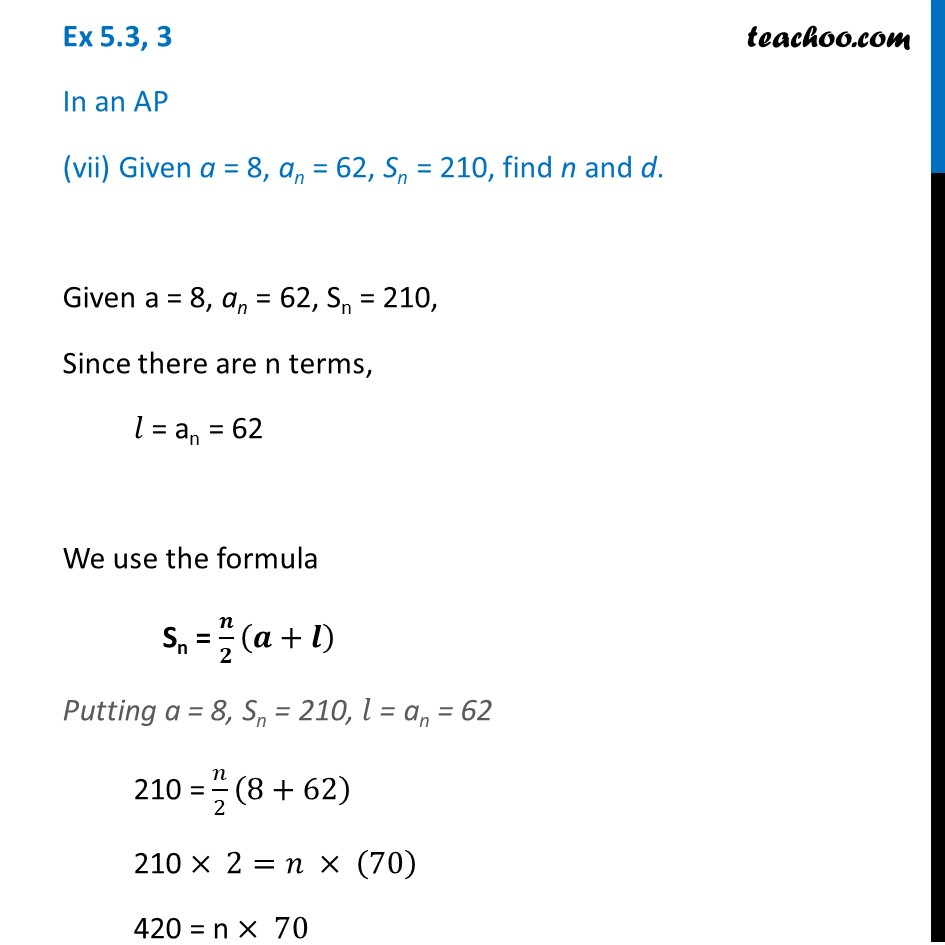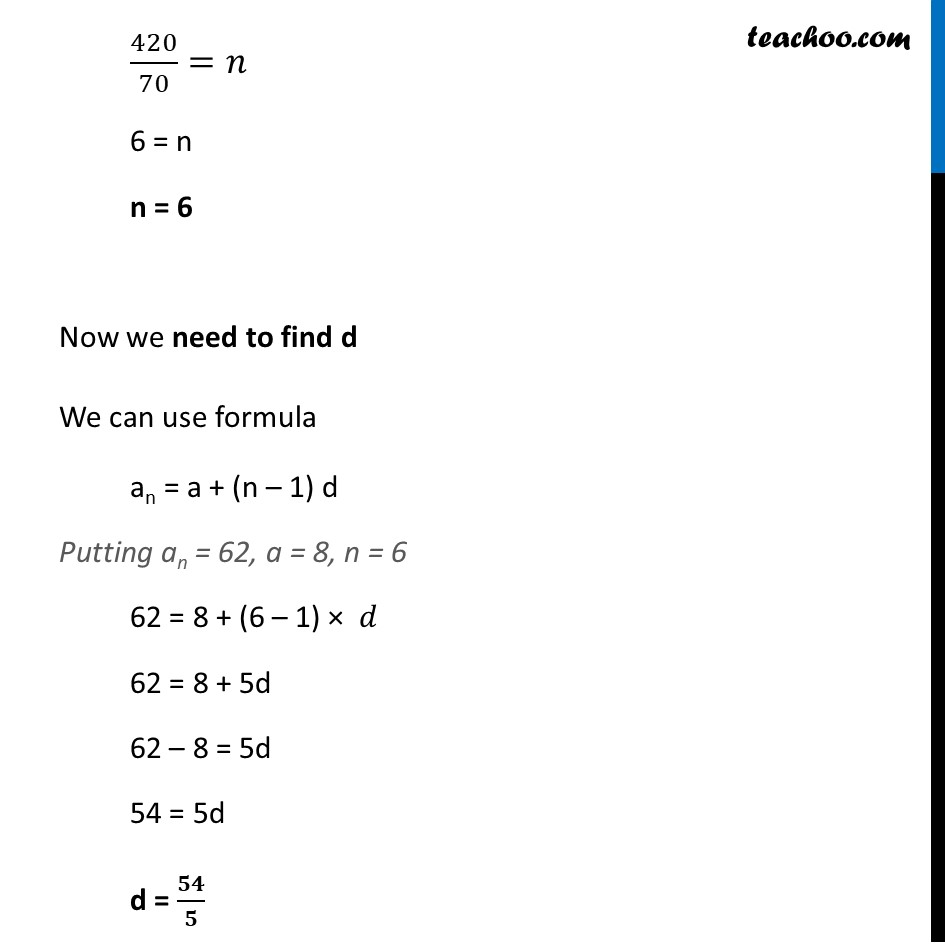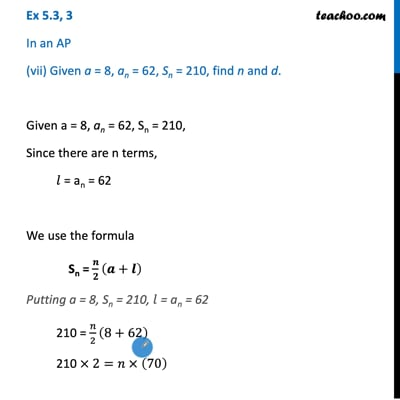Ex 5.3

Chapter 5 Class 10 Arithmetic Progressions (Term 2)
Serial order wiseThis video is only available for Teachoo black users

### Transcript

Ex 5.3, 3 In an AP (vii) Given a = 8, an = 62, Sn = 210, find n and d. Given a = 8, an = 62, Sn = 210, Since there are n terms, 𝑙 = an = 62 We use the formula Sn = 𝒏/𝟐 (𝒂+𝒍) Putting a = 8, Sn = 210, 𝑙 = an = 62 210 = 𝑛/2 (8+62) 210 × 2=𝑛 × (70) 420 = n × 70 420/70=𝑛 6 = n n = 6 Now we need to find d We can use formula an = a + (n – 1) d Putting an = 62, a = 8, n = 6 62 = 8 + (6 – 1) × 𝑑 62 = 8 + 5d 62 – 8 = 5d 54 = 5d d = 𝟓𝟒/𝟓